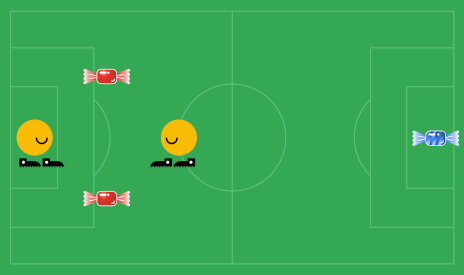시간 제한메모리 제한제출정답맞힌 사람정답 비율
서브태스크 참고 (추가 시간 없음) 1024 MB306621.429%

## 문제

Mr. Jolly teaches football (or soccer, for US speakers) to $\mathbf{N}$ children numbered from $1$ to $\mathbf{N}$. He has taken to leaving sweets on the field where the games take place, one for each child. After the game is finished, each child can grab and eat one sweet as their reward.

The children are tired after games, so each child wants to grab the sweet closest to them (using Euclidean distance). This could lead to fights — if the same sweet is closest to two or more children. To avoid that, after the game all the children stop where they are, and Mr. Jolly calls out their names, one by one. When a child's name is called, they grab the closest sweet to them (out of the ones that weren't already grabbed, of course). In the case where two or more sweets are tied for the smallest distance, Mr. Jolly can decide which one the child grabs.This has worked very well for Mr. Jolly for a while now, but today disaster struck! While laying out the sweets, Mr. Jolly accidentally dropped his blueberry jelly that he planned to eat after all the children go home. So now there are $\mathbf{N}$ children on the field, and $\mathbf{N}+1$ sweets. The sweets are numbered from $1$ to $\mathbf{N} + 1$, with sweet $1$ being Mr. Jolly's blueberry jelly. Is there a way for Mr. Jolly to save his blueberry jelly by calling the children's names in such an order that the blueberry jelly is the one sweet left over?

## 입력

The first line of the input gives the number of test cases, $\mathbf{T}$. $\mathbf{T}$ test cases follow. Each test begins with a line containing a single integer, $\mathbf{N}$, the number of children on the field. The next $\mathbf{N}$ lines describe the positions of the children. Each of these lines contains two integers, $\mathbf{X_i}$ and $\mathbf{Y_i}$, representing the position of the $i$⁠-th child after the game ends. Then there are $\mathbf{N}+1$ more lines that describe the positions of sweets after the game, where the first of the sweets is Mr. Jolly's blueberry jelly. Each of these lines contains two integers, $\mathbf{X_j}$ and $\mathbf{Y_j}$, representing the position of the $j$⁠-th sweet.

## 출력

For each test case, output one line containing Case #x: y, where $x$ is the test case number (starting from 1) and $y$ is IMPOSSIBLE if there is no way Mr. Jolly can choose the children (and break ties for the closest sweet) to leave his blueberry jelly uneaten. Otherwise, if Mr. Jolly can save his blueberry jelly, $y$ is POSSIBLE. If Mr. Jolly can save his jelly, output $\mathbf{N}$ additional lines representing the order the children will go and which jellies they will pick. The $i$⁠-th line should contain two integers $A_i$ and $B_i$ representing that child $A_i$ will go next and will pick sweet $B_i$. The sweet $B_i$ must be the closest (or tied for the closest) sweet to child $A_i$ when they go to pick their sweet.

## 제한

• $1 \le \mathbf{T} \le 100$.
• $-10^9 \le \mathbf{X_i} \le 10^9$, for all $i$.
• $-10^9 \le \mathbf{Y_i} \le 10^9$, for all $i$.
• $-10^9 \le \mathbf{X_j} \le 10^9$, for all $j$.
• $-10^9 \le \mathbf{Y_j} \le 10^9$, for all $j$.

## Test Set 1 (10점)

시간 제한: 10 초

• $1 \le \mathbf{N} \le 10$.

## Test Set 2 (18점)

시간 제한: 45 초

• $1 \le \mathbf{N} \le 1000$.

## 예제 입력 1

4
2
-3 0
-1 0
3 0
-2 -1
-2 1
1
0 0
1 1
2 2
3
10 0
-10 0
0 0
0 5
-1 0
5 0
0 -5
2
3 4
3 4
5 7
3 4
5 7


## 예제 출력 1

Case #1: POSSIBLE
2 2
1 3
Case #2: IMPOSSIBLE
Case #3: POSSIBLE
3 2
2 4
1 3
Case #4: POSSIBLE
1 2
2 3


## 힌트

Sample Case #1 is illustrated in the image above. Notice that each child is equally close to each of the two non-blueberry-jelly sweets. In our solution, Mr. Jolly assigns the second sweet to the second child and the third sweet to the first child, successfully leaving the first sweet (the blueberry jelly) for himself.

In Sample Case #2, the sole child is closer to the blueberry jelly than to the other sweet, so Mr. Jolly cannot prevent his precious blueberry jelly from being eaten.

In Sample Case #3, we present one of many solutions; it is actually possible to call the children in any order.

In Sample Case #4, note that children might share the same position, sweets might share the same position, and children and sweets might share the same position.

## 채점 및 기타 정보

• 예제는 채점하지 않는다.